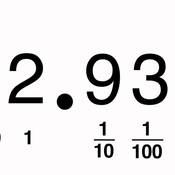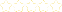• > Rachel Casto
• > Decimal Notation and Decimal Fractions
+# Decimal Notation and Decimal Fractions

Owner: Rachel Casto
##### Description:
Number and Operations—Fractions 4.NF  C. Understand decimal notation for fractions, and compare decimal fractions. 5. Express a fraction with denominator 10 as an equivalent fraction with denominator 100, and use this technique to add two fractions with respective denominators 10 and 100. 4 For example, express 3/10 as 30/100, and add 3/10 + 4/100 = 34/100. NOTE: 4Students who can generate equivalent fractions can develop strategies for adding fractions with unlike denominators in general. But addition and subtraction with unlike denominators in general is not a requirement at this grade. 6. Use decimal notation for fractions with denominators 10 or 100. For example, rewrite 0.62 as 62/100; describe a length as 0.62 meters; locate 0.62 on a number line diagram. 7. Compare two decimals to hundredths by reasoning about their size. Recognize that comparisons are valid only when the two decimals refer to the same whole. Record the results of comparisons with the symbols >, =, or <, and justify the conclusions, e.g., by using a visual model.  NOTE: Grade 4 expectations in this domain are limited to fractions with denominators 2, 3, 4, 5, 6, 8,10, 12, and 100. Measurement and Data ⎯ 4.MD  A. Solve problems involving measurement and conversion of measurements from a larger unit to a smaller unit.  2. Use the four operations to solve word problems involving distances, intervals of time, liquid volumes, masses of objects, and money, including problems involving simple fractions or decimals, and problems that require expressing measurements given in a larger unit in terms of a smaller unit. Represent measurement quantities using diagrams such as number line diagrams that feature a measurement scale.
3 Tutorials in this Playlist

#### Decimal-Fraction Equivalencies

Author: Rachel Casto
Rating:(0)

#### Comparing Decimals

Author: Rachel Casto
Rating:(0)